# Spheres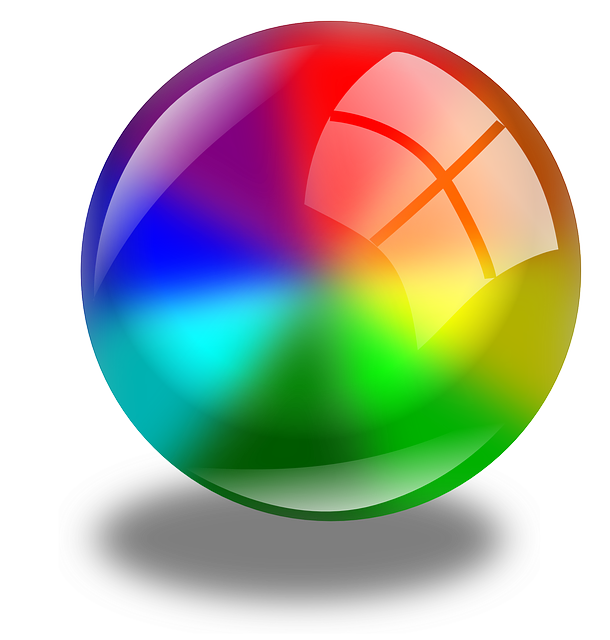A sphere is a solid object that

• is perfectly round
• has one curved surface
• has no edges or vertices
• is perfectly symmetrical.

Every point on the surface of a sphere is the same distance (called the radius and denoted by $r$ ) away from the centre of the sphere. A sphere is basically a 3-dimensional analogue of a circle. When you slice through a sphere, you get sections shaped like circles.

Spheres are not polyhedrons as they do not have flat faces, but only a single curved surface.## Examples of Spheres

### Balls and Marbles

Balls and marbles are shaped like spheres so that they roll nicely.

For example, this cricket ball is shaped like a sphere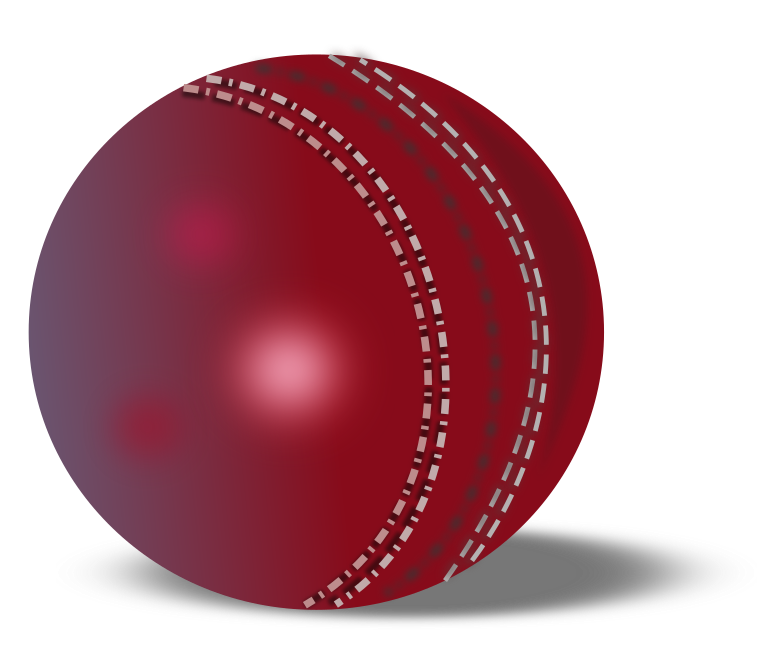and so are these glass marbles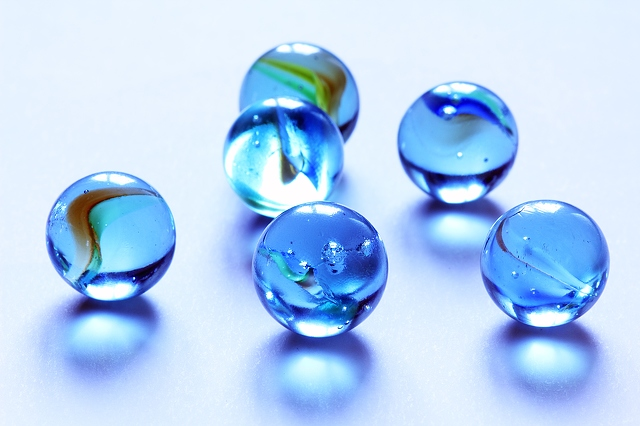Balloons try to approximate a spherical shape as much as possible as they are inflated.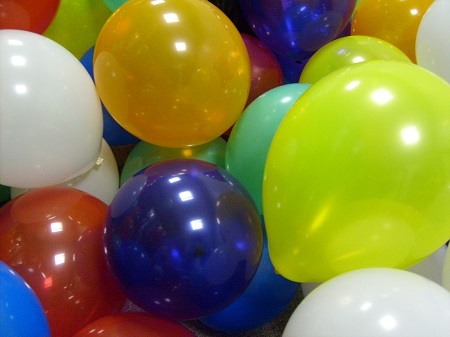### Spheres in Nature

The ratio of the surface area of a sphere to its volume is the smallest of any geometric shape. As a result, some objects in nature try to take on a spherical shape.

Bubbles and water drops have spherical shapes as seen in the picture below.Planets and stars have shapes that closely resemble spheres. For example, our planet, Earth is very nearly a sphere, except that it's a bit squashed at the north and south poles. We call it a spheroid because it is only different from a sphere in one direction.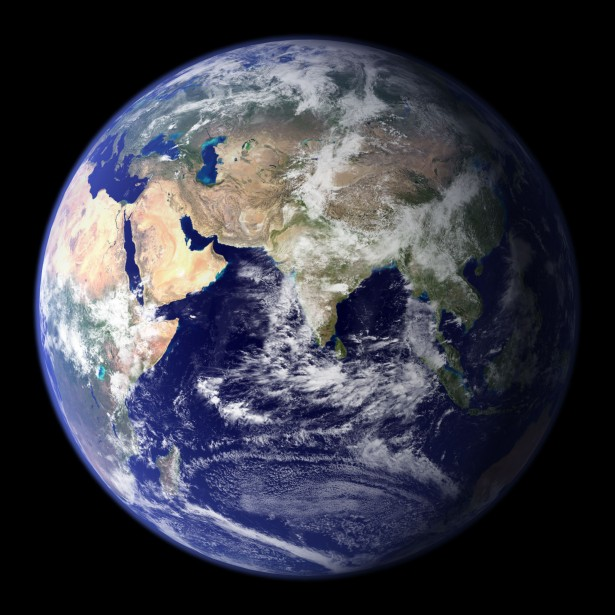The Sun is said to be the closest approximation to a sphere in nature: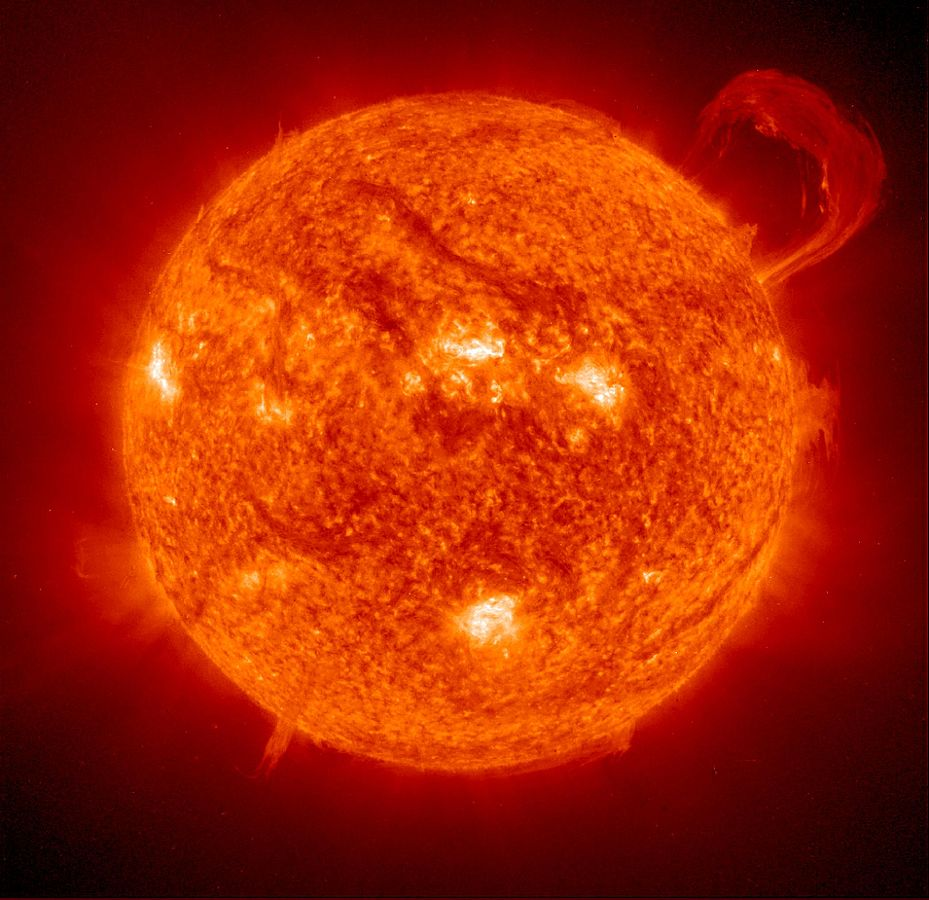## The Surface Area and Volume of a SphereThe surface area and volume of a sphere are given by the following formulas:

\begin{align*} V &= \dfrac{4\pi}{3} r^3\\ SA &= 4 \pi r^2, \end{align*}
where $r$ is the radius. If you've done some Calculus, you might notice that the formula for the surface area of a sphere is the derivative of the formula for the volume of a sphere with respect to $r$. If you haven't, completely ignore that last remark.

For example, if a sphere has radius $r = 4$ cm, then its volume is given by

$V = \dfrac{4}{3} \times \pi \times 4^3 = \dfrac{256}{3}\; \pi \approx 268.1 \text{ cm}^3$,
and its surface area is given by
$SA =4 \times \pi \times 4^2 =64 \pi \approx 201.06 \text{ cm}^2$.

The ratio between the surface area and volume of the sphere of radius $4$ cm is then $201.06: 268.1$, which is about $3: 4$.

This is a comparatively small surface area to volume ratio.

### Some Other Spherical Terms

The largest circular slice you can take through a sphere is called its great circle. This is any circular slice through the centre of the sphere.

The two halves of a sphere that are formed when it is sliced throught its centre are called hemispheres. I think you might have heard that term before.

### Description

There are several lessons related to 3D geometry such as

1. Euler's formula
2. Vertices, Edges and Faces
3. Volumes of 3D shapes
4. etc

Even though we've titled this lesson series to be more inclined for Year 10 or higher students, however, these lessons can be read and utilized by lower grades students.

### Prerequisites

Understanding of 3D shapes

### Audience

Year 10 or higher, but suitable for Year 8+ students

### Learning Objectives

Get to know 3D Geometry

Author: Subject Coach
You must be logged in as Student to ask a Question.